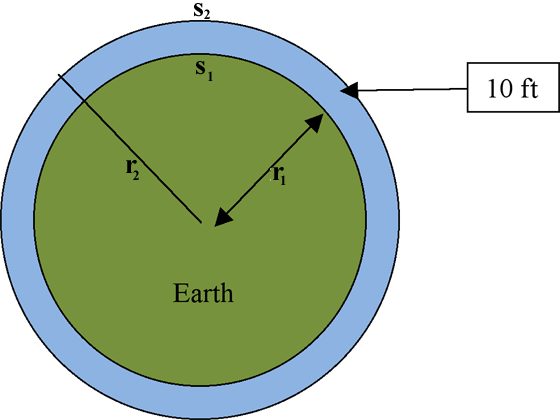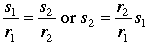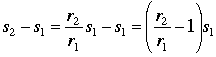SEARCH HOMEMath Central Quandaries & QueriesQuestion from grant, a parent: if you have 2 plumb structures 10ft. tall, how far apart will they be when they are 1 inch out of parallel at the topHello,
We assume that the Earth is a perfect sphere (which it is not) and look at the cross section determined by these two  10 ft  structures:Now we use radian measure, namely the equation:  θ = s/r

Since these structures are plumb, they lie perfectly on the radial lines of the Earth.  Thus,  θ is the same for the small and big circles, and we obtain:Therefore the difference between these two arc lengths is,So we wish to solve for s1 knowing that s2 - s1 = 1 inch = 1/12 foot, and r1 = r2 + 10 feet.

What is the radius of the Earth in feet?  How far apart are the two structures?
TylerMath Central is supported by the University of Regina and The Pacific Institute for the Mathematical Sciences.